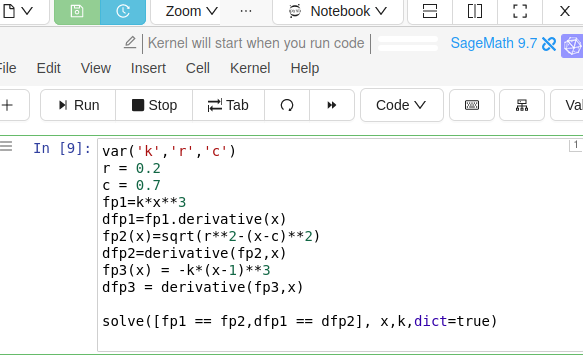# solving 2 non-linear functions (max degree of 3) fails

I'm having trouble solving the following:It's a simple system that uses the functions and their respective derivatives to find values fo x and k. Mathematica has no problem with this. My machine and the cocalc machine become compute bound. My machine runs out of memory. How do I redo this to get sage to solve it ? Should I try a numeric solve ?

edit retag close merge delete

Sort by » oldest newest most voted

Un gros MERCI Emmanuel! I got the solution I needed for the 3rd part of this piecewise funtion (it agrees with mathematica) I need the k and the x values that are real values:

 *****50.4900997166127
0.881173769148990*****


Here is the code. It took a little bit of digging to get the substitutions. It would also be nice to know if there is an option in solve to just return the real values :)

  fp2 = sqrt(r^2-(x-c)^2)
dfp2 = derivative(fp2, x)
fp3 = -k*(x-1)^3
dfp3 = derivative(fp3, x)
sys = [fp2 == fp3,dfp2 == dfp3]
SS = solve(sys, (x, k), algorithm="sympy")
print(SS[k].subs(c=0.7,r=0.2))
print(SS[x].subs(c=0.7,r=0.2))
print(SS[k].subs(c=0.7,r=0.2))
print(SS[x].subs(c=0.7,r=0.2))

*****
50.4900997166127
0.881173769148990
*****
1.04977199683675*I
0.368826230851010


​Bien à vous! Pat Browne Rimouski Québec

more

Edited for legibility.

You can select the all-real solutions directly from the symbolic solution :

sage: [s for s in [dict(zip(v.keys(), [u.subs({r:1/5, c:7/10}) for u in v.values()])) for v in SS] if all([t in AA for t in s.values()])]
[{k: -128*sqrt(-1/100*(5*sqrt(29/5) - 7)^2 + 16/25)/(sqrt(29/5) - 7)^3,
x: -1/8*sqrt(29/5) + 7/8}]


or, with "float" approximations :

sage: [s for s in [dict(zip(v.keys(), [CDF(u.subs({r:1/5, c:7/10})) for u in v.values()])) for v in SS]
if all([t in RDF for t in s.values()])]
[{k: 0.8212757749910893, x: 0.5739601355301926}]


Note : I tend to use exact values (symbolic or rational) rather than inexact values ("float" or RR/RDF) in explicit solutions (thus avoiding the problems of comparing floats values).

Note that these two special solutions allow to check easily that fp3 != dfp3 :

sage: [(fp3-dfp3).subs(s).subs({r:1/5, c:7/10}).is_zero() for s in SS]
[False, False]
sage: [(fp3-dfp3).subs(s).subs({r:1/5, c:7/10}).n() for s in SS]
[0.510718865288295, 1.42320478260899e-18 + 0.0232426979533997*I]


Merci encore, il y a toujours un moyen... il faut s'habituer aux objets comme AA, RR,RDF :)

Sage's default solver (i. e. Maima's) indeed fails to solve your system. But Sympy's does. After running :

var('k, c, r')
# Keep these constant symbolic, for clarity's and generality's sake...
# r = 0.2
# c = 0.7
# r = 1/5
# c = 7/10
fp1 = k*x^3
dfp1 = fp1.derivative(x)
fp2 = sqrt(r^2-(x-c)^2)
dfp2 = derivative(fp2, x)
fp3 = -k*(x-1)^3
# Unused for now...
dfp3 = derivative(fp3, x)
sys = [fp1 == fp2, dfp1 == dfp2]


we get two solutions :

sage: SS = solve(sys, (x, k), algorithm="sympy") ; SS
[{k: 16*sqrt(-(c - sqrt(c^2 + 24*r^2))^2 + 16*r^2)/(5*c - sqrt(c^2 + 24*r^2))^3,
x: 5/4*c - 1/4*sqrt(c^2 + 24*r^2)},
{k: 16*sqrt(-(c + sqrt(c^2 + 24*r^2))^2 + 16*r^2)/(5*c + sqrt(c^2 + 24*r^2))^3,
x: 5/4*c + 1/4*sqrt(c^2 + 24*r^2)}]


Checking these solutions isn't absolutely trivial, Sage having simplification problems with radical expressions :

sage: [all([bool(u.subs(s)) for u in sys]) for s in SS]
[False, False]


But we can check that the difference of left- and right-hand sides of each equation is indeed null for each solution :

sage: [all([(u.lhs()-u.rhs()).subs(s).canonicalize_radical().is_zero() for u in sys]) for s in SS]
[True, True]


Solving and checking in Mathematica is left to the reader as an exercise... Also left to the reader is to check that none of these solutions satisfies fp3 == dfp3...

HTH,

more